Ex 11.4

Chapter 11 Class 11 Conic Sections
Serial order wise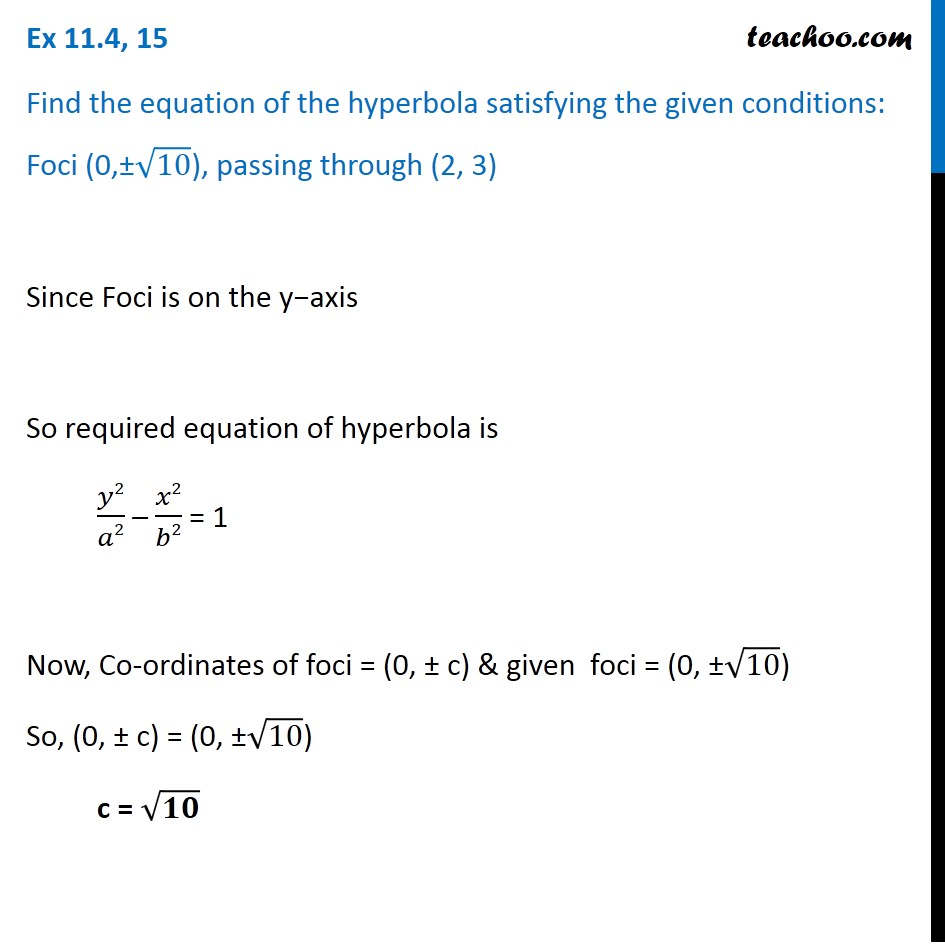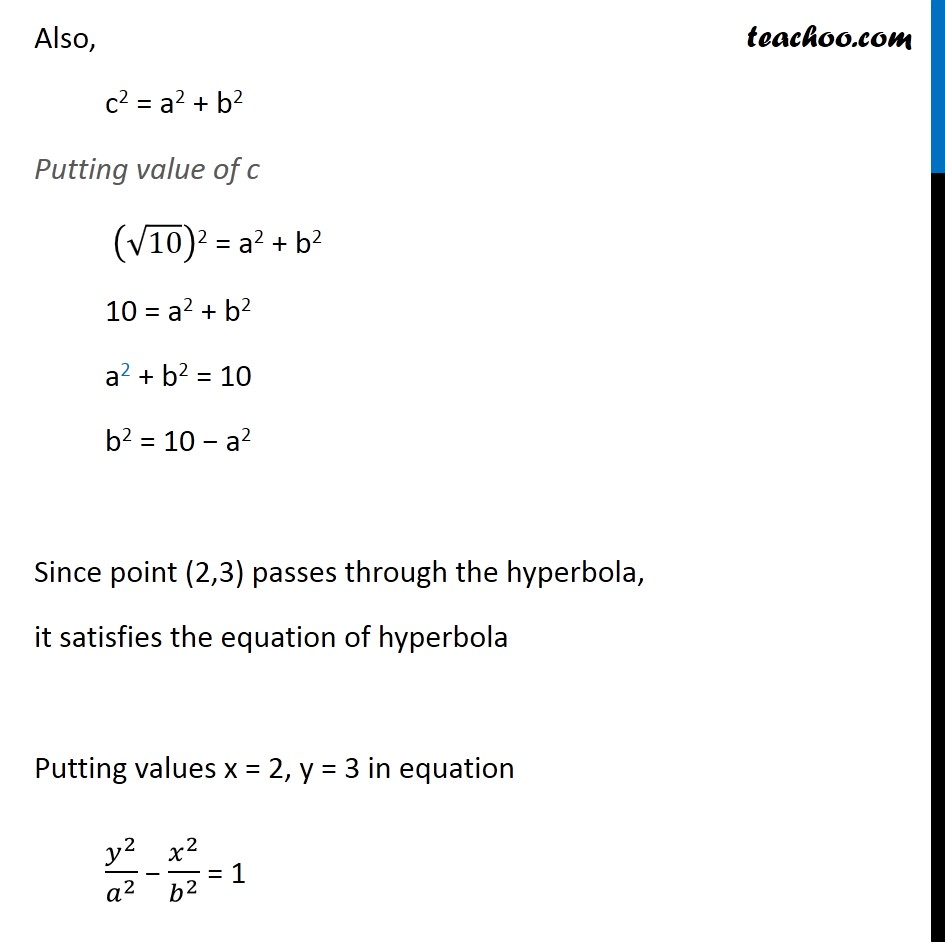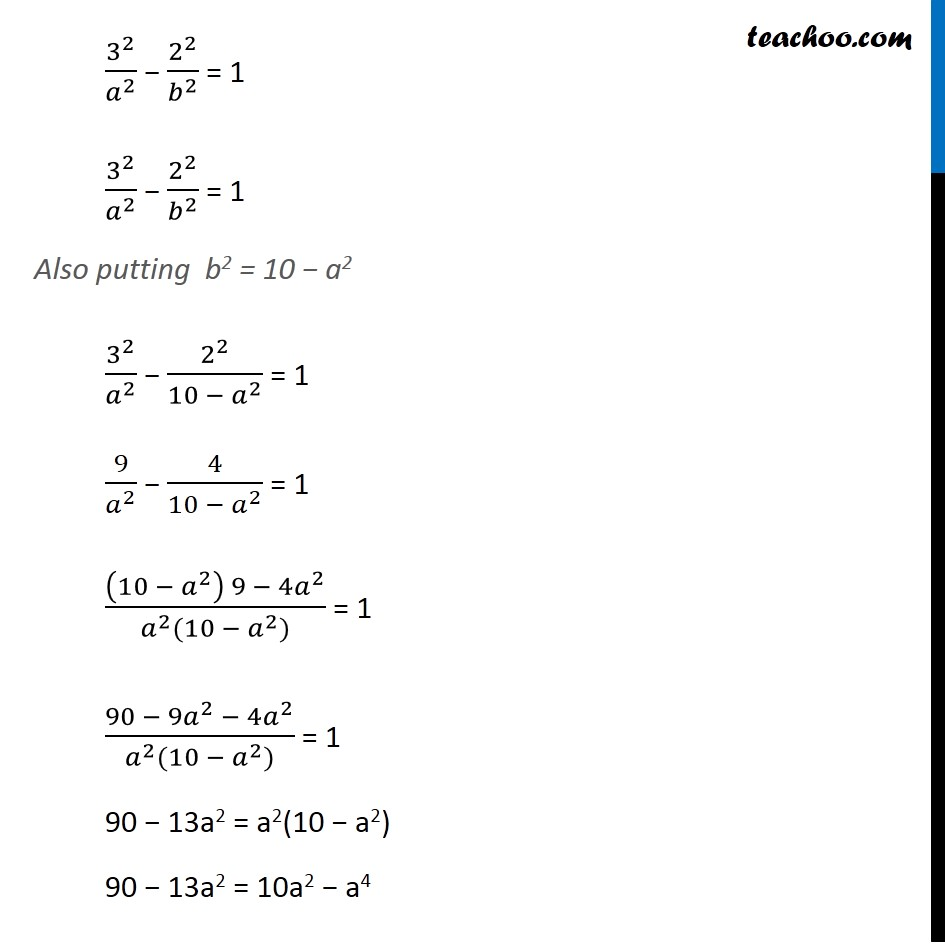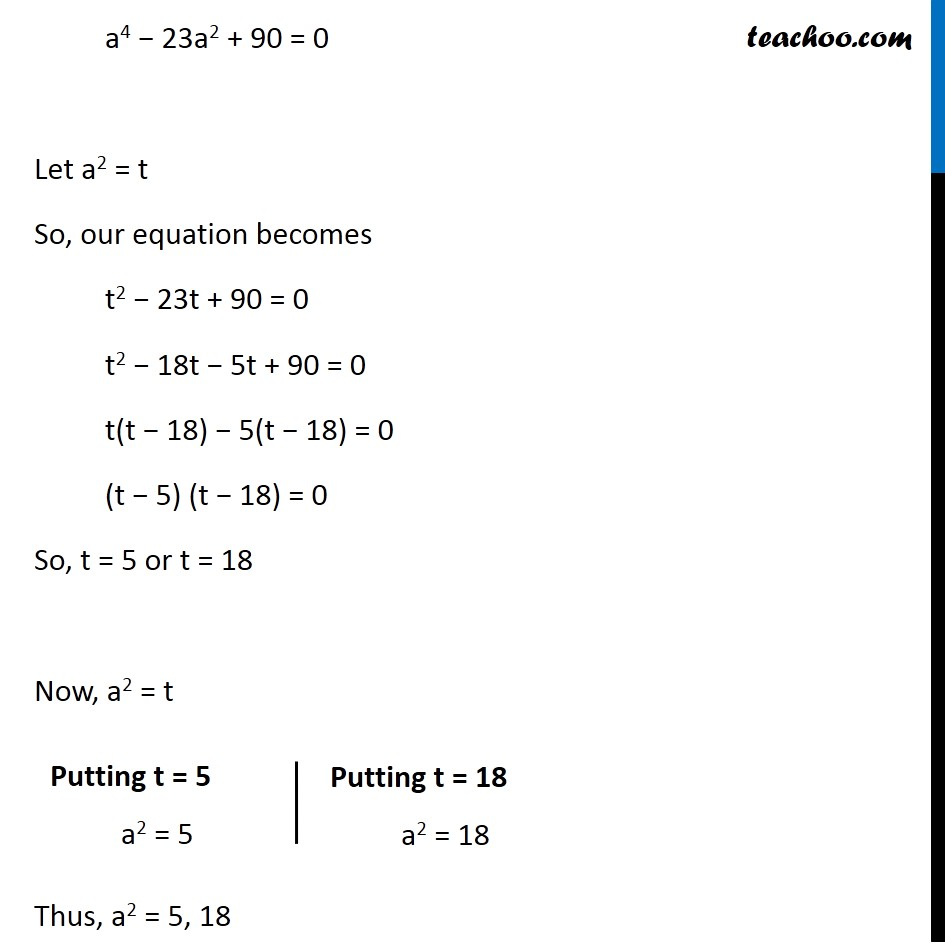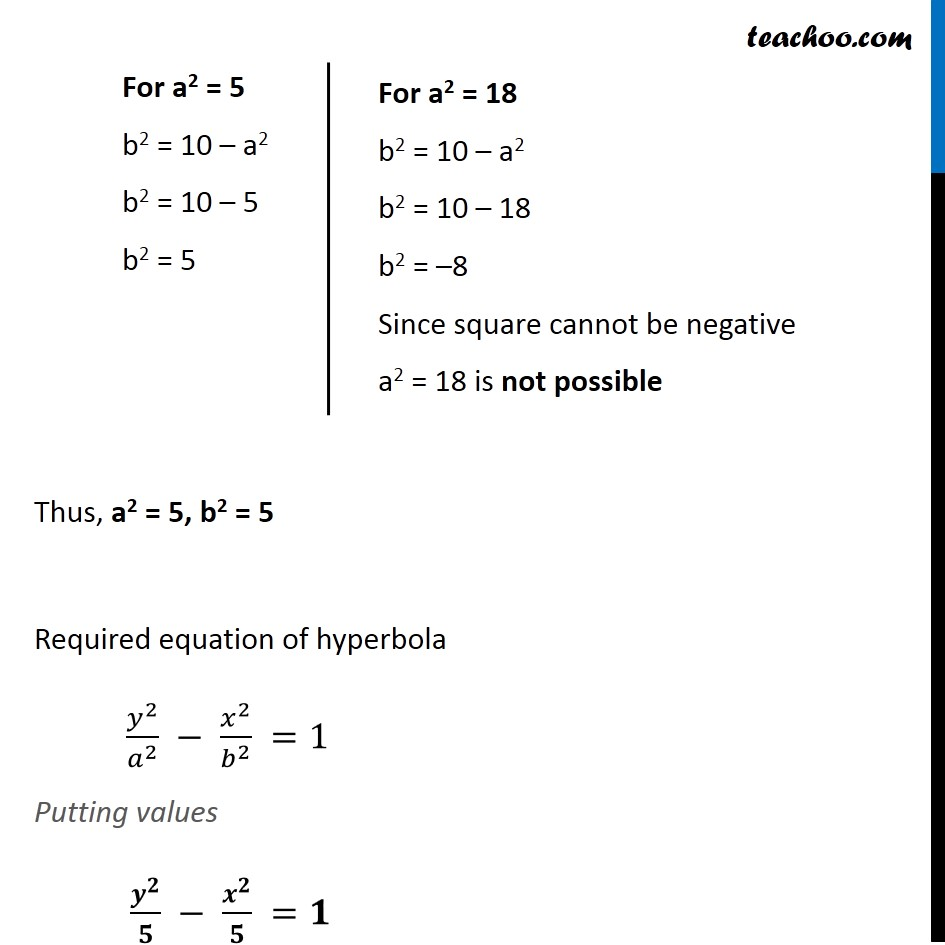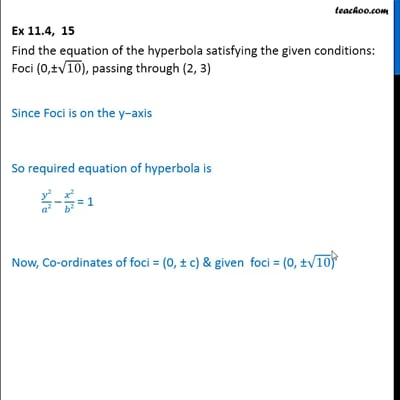This video is only available for Teachoo black users

Solve all your doubts with Teachoo Black (new monthly pack available now!)

### Transcript

Ex 11.4, 15 Find the equation of the hyperbola satisfying the given conditions: Foci (0,±√10), passing through (2, 3) Since Foci is on the y−axis So required equation of hyperbola is 𝑦2/𝑎2 – 𝑥2/𝑏2 = 1 Now, Co-ordinates of foci = (0, ± c) & given foci = (0, ±√10) So, (0, ± c) = (0, ±√10) c = √𝟏𝟎 Also, c2 = a2 + b2 Putting value of c (√10)2 = a2 + b2 10 = a2 + b2 a2 + b2 = 10 b2 = 10 − a2 Since point (2,3) passes through the hyperbola, it satisfies the equation of hyperbola Putting values x = 2, y = 3 in equation 𝑦^2/𝑎^2 − 𝑥^2/𝑏^2 = 1 a 3^2/𝑎^2 − 2^2/𝑏^2 = 1 3^2/𝑎^2 − 2^2/𝑏^2 = 1 Also putting b2 = 10 − a2 3^2/𝑎^2 − 2^2/(10 − 𝑎^2 ) = 1 9/𝑎^2 − 4/(10 − 𝑎^2 ) = 1 ((10 − 𝑎^2 ) 9 − 4𝑎^2)/(𝑎^2 (10 − 𝑎^2)) = 1 (90 − 9𝑎^2 − 4𝑎^2)/(𝑎^2 (10 − 𝑎^2)) = 1 90 − 13a2 = a2(10 − a2) 90 − 13a2 = 10a2 − a4 a4 − 23a2 + 90 = 0 Let a2 = t So, our equation becomes t2 − 23t + 90 = 0 t2 − 18t − 5t + 90 = 0 t(t − 18) − 5(t − 18) = 0 (t − 5) (t − 18) = 0 So, t = 5 or t = 18 Now, a2 = t Thus, a2 = 5, 18 Putting t = 5 a2 = 5 Putting t = 18 a2 = 18 For a2 = 5 b2 = 10 – a2 b2 = 10 – 5 b2 = 5 For a2 = 18 b2 = 10 – a2 b2 = 10 – 18 b2 = –8 Since square cannot be negative a2 = 18 is not possible Thus, a2 = 5, b2 = 5 Required equation of hyperbola 𝑦^2/𝑎^2 − 𝑥^2/𝑏^2 =1 Putting values 𝒚^𝟐/𝟓 − 𝒙^𝟐/𝟓 =𝟏